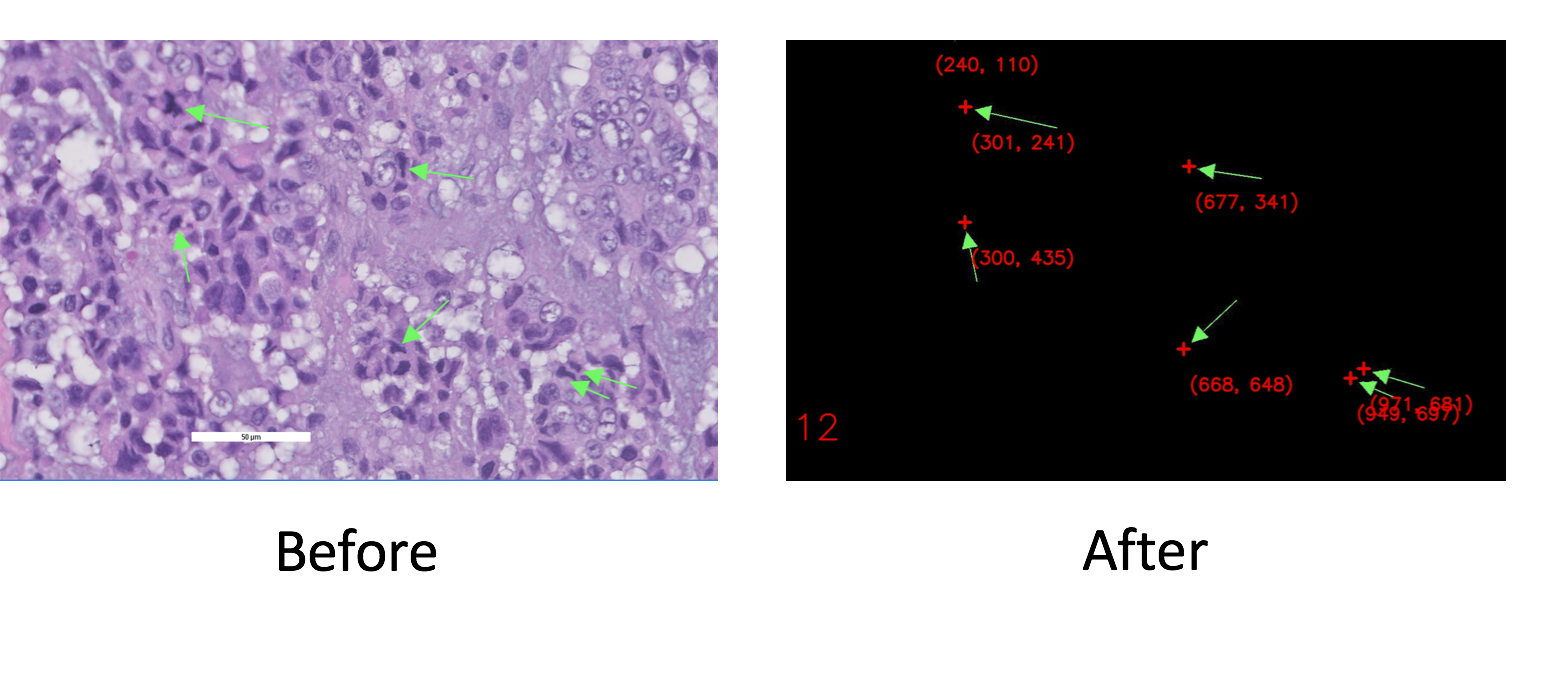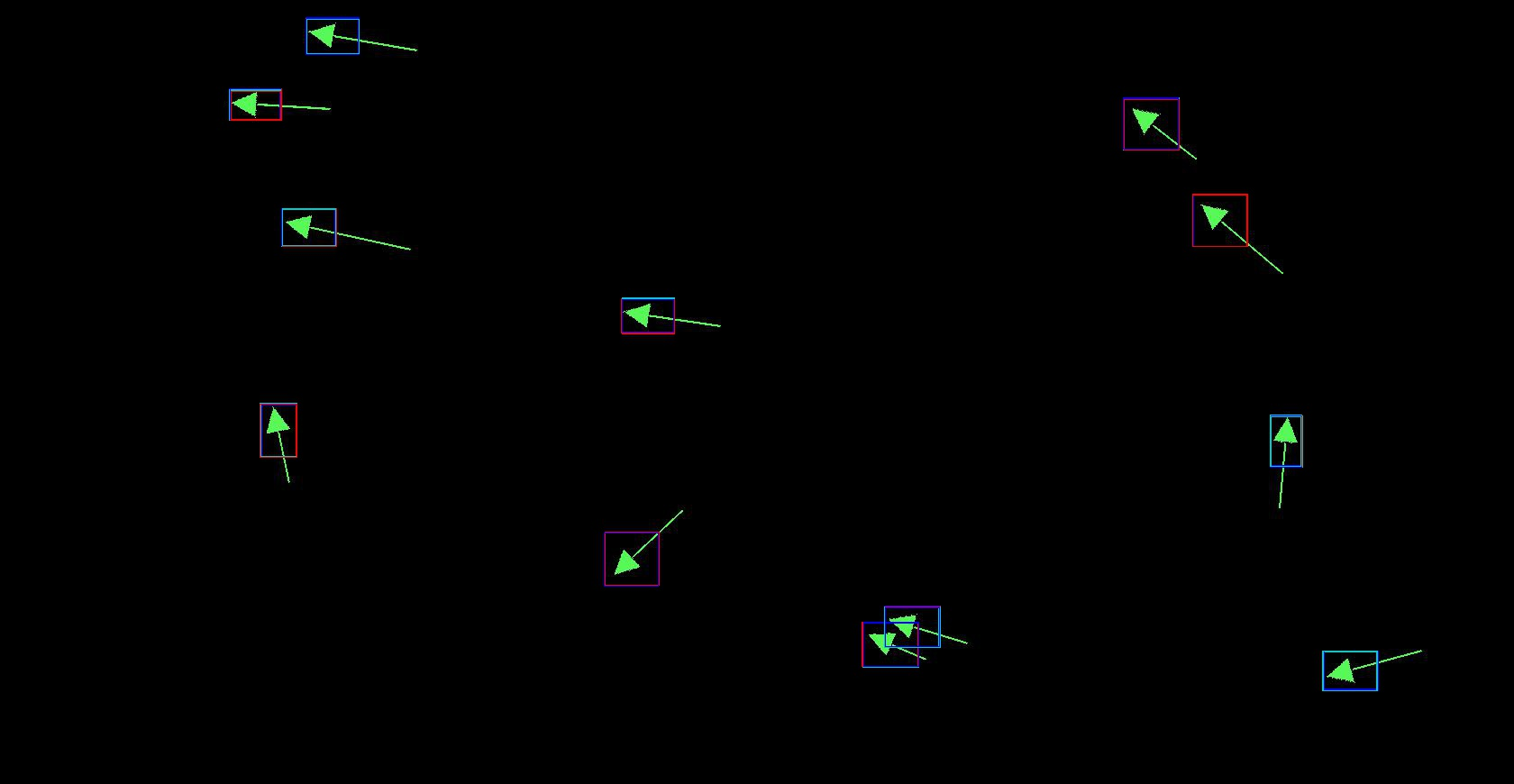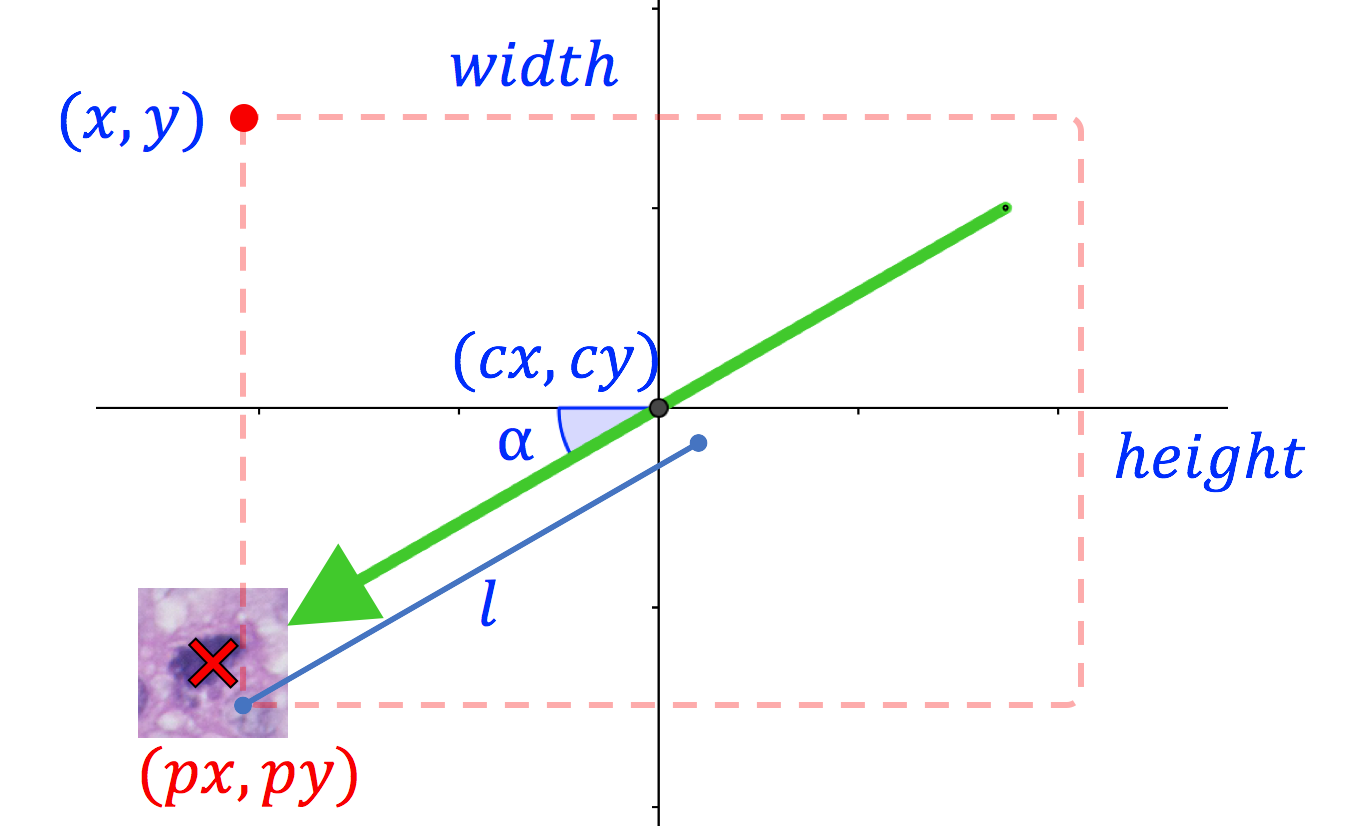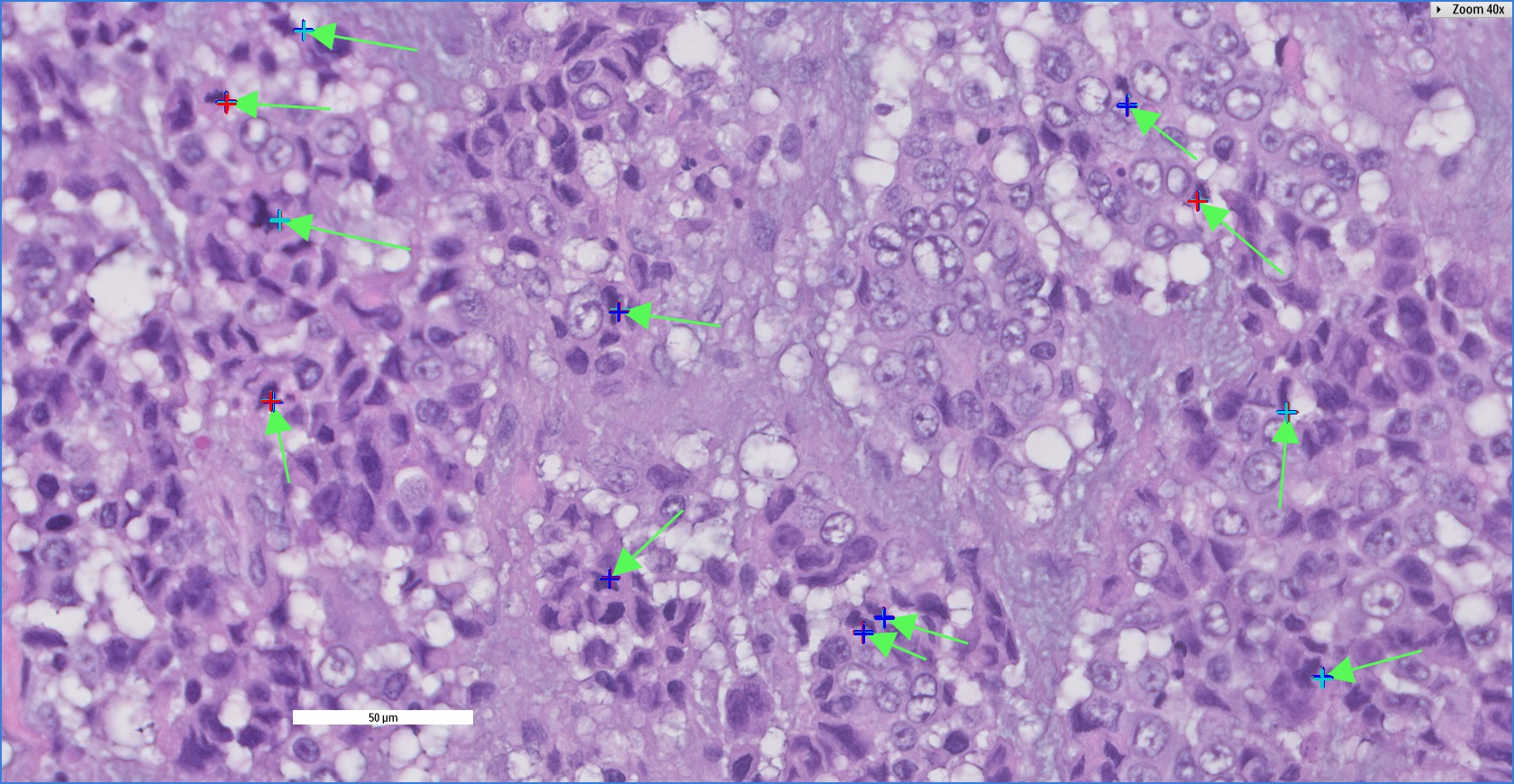# Mitosis Image Processing Part 2 - Locating Final Points of InterestThe problem we are trying to solve is find the exact locations of cells undergoing mitosis in histology images. These are the cells that are annotated with green arrows. In other words, given the Before image above, we want to extract data shown in After.

In Part 1, I have detailed how I used template matching in openCV to obtain bounding boxes on the arrows with 100% accuracy. In this post, I will describe my algorithm used to transform the bounding box information into the coordinates of the arrowheads.

## The Input Data

We use the code in Part 1, but with one important change:

import numpy as np
import cv2
import os
from scipy import misc, ndimage
from multiprocessing import Pool
import pickle
import math
import re
import csv

PKL_DIR = {path to pickle files}
LOC_DIR = {path to final csv files}

{import functions from Part 1}

def match_and_pickle(img_name):
""" Apply template matching to img_name in IMG_DIR, using

Output: Coordinates of the top left corner of matching templates,
in the format [(deg, [points])], saved in img_name.pkl.
"""
print('Matching img %s' %img_name)
img_gray = cv2.cvtColor(img_rgb, cv2.COLOR_BGR2GRAY)
matches = []
for deg in range(0, 360, MATCH_RES):
if tmpl is None or mask is None:
print('Failed to match for tmpl %d.' %deg)
else:
w, h = tmpl.shape[::-1]
loc = np.where(res < MATCH_THRESH)
pts = zip(*loc[::-1])   #top left corners of arrow bounding box
if len(pts)>0:
matches.append((deg, pts))
file_name = os.path.join(PKL_DIR,
"".join(img_name.split('_')[:-1])+'.pkl')  #eg M21.pkl
with open(file_name,'wb') as f:
pickle.dump(matches, f)
return


Here, I replaced the function match_and_draw() from Part 1 with match_and_pickle(). The difference is that instead of drawing the bounding boxes the images, we save the location the arrows and their orientations as pickled data.

Recall that we have 360 arrow templates, one for each degree of orientation, with which we perform template matching. For each image, we save the data in the following Python tuple list format:
[(deg, [(x, y), ...]), ...], where deg is the orientation of the template that matched, and (x, y) are the coordinates of the matching bounding box. Note that these are the positions of the top left corners of the bounding boxes.

I can visualize then the bounding boxes with the following code, which loads the pickle data generated by match_and_pickle() and draws the bounding boxes on a given image.

class Color_Iterator(object):
colors = [(0,0,255), (255,0,0), (204,204,0)]
i = 0

def next(self):
self.i += 1
return self.colors[self.i % len(self.colors)]

CI = Color_Iterator()

def draw_from_pkl(img_name, output_path='./'):
""" Draws bounding boxes on stripped ver of img_name with matches from
img_name.pkl.
Saves output image in output_path.

Example: draw_from_pkl(M21.jpg, './') draws boxes on M21_s.jpg, and
saves output as M21_r.jpg in the current directory.
"""
img_name = ''.join(img_name.split('.')[:-1])+'_s.jpg'
pkl_name = os.path.join(PKL_DIR,
"".join(img_name.split('_')[:-1])+'.pkl')  #eg M21.pkl
with open(pkl_name,'r') as f:
for (deg, pts) in matches:
w, h = tmpl.shape[::-1]
for pt in pts:
cv2.rectangle(img_rgb, pt, (pt + w, pt + h), CI.next(), 1)
img_name = "".join(img_name.split('_')[:-1])
cv2.imwrite(os.path.join(output_path, img_name+'_r.jpg'), img_rgb)  #eg M21_r.jpg


The above code produces images that appear like so:Here, I used Color_Iterator to iterate the color of the boxes drawn. This is to highlight an important feature: There are multiple matches for each arrow. This is because two templates a degree apart may both match on the same arrow; or one template could match many times on the same arrow, each a few pixels apart.

This fact is important later on because it means that there is a many-to-one mapping from matches to arrows. Since we only want one point per arrow in our final output, we need to coalesce a bunch of matches per arrow into one single point.

## Geometric Transformation and Coalescing Algorithm

### Transform

One important question is still unanswered. Currently, we only have information regarding the location of the top left corner of the bounding boxes. As such, how do we transform these points into the position of the arrowheads? With the orientation of the arrows retrieved from our matching algorithm, this can be done with simple trigonometry!Consider the above schematic. The dashed box represents the bounding box on the arrow. $$\alpha$$, $$(x, y)$$, $$width$$ and $$height$$ are known quantities, where $$\alpha$$ is the rotation of the matching template, and $$width$$, $$height$$ are the dimensions of the bounding box.

The center of the bounding box $$(cx, cy)$$, which is also the center of the arrow, is:

$(cx, cy) = (x+\frac{width}{2}, y+\frac{height}{2})$

Then, the quantities we are after, $$(px, py)$$, are:

$px = cx - l \cos(\alpha)$ $py = cy + l \sin(\alpha)$

where $$l$$ is the practical pixel radius of the arrow. It is longer than the actual half-length of the arrows because we want $$(px, py)$$ to be slightly ahead of the arrow tip.

Note that in this case, I have defined the negative x-axis to be zero degrees, and the angle increases anti-clockwise.

RADIUS = 42

def transform(matches):
""" Transforms a list of (deg * coordinate list), where the coordinates
are of the top-left corner of the tmpl bounding boxes, to a list containting
the coordinates of the tips of the arrows.
Returns the list of transformed coordinates.
"""
tips = []
for (deg, pts) in matches:
tmpl = cv2.imread(os.path.join(TMPL_DIR, 'tmpl%d.png' %deg), 0)
w, h = tmpl.shape[::-1]
for pt in pts:   #top left corner of arrow bounding box
(cx, cy) = (pt+w/2, pt+h/2)  #center of the arrow
return tips


In the above code,

• The parameter matches is the list generated by match_and_picke()
• $$\alpha=$$ deg
• $$(x, y)=$$ pt
• $$width=$$ w
• $$height=$$ h
• $$l=$$ RADIUS
• $$[(px, py), ...]=$$ tips

Plotting the list of $$(px, py)$$ on the original image will give us something like this:Again, if you observe closely, there are multiple points per arrow.

### Coalesce

To produce our final output, coalescing multiple points into one final point is straightforward: if two points $$a$$ and $$b$$ are within a certain distance, then $$b$$ is a duplicate.

def coalesce(tips):
""" Reduces points close to together in tips into one point.
Returns the list of reduced points.
"""
thresh = 7

def not_distinct(p1, p2):
(p1x, p1y) = p1
(p2x, p2y) = p2
return (p1x-p2x)**2 + (p1y-p2y)**2 <= thresh**2

def update(pt, distinct_pts):
for dpt in distinct_pts:
if not_distinct(pt, dpt):
return
distinct_pts.append(pt)
return

if len(tips) <= 1:
return tips
distinct_pts = []
for pt in tips:
update(pt, distinct_pts)
return distinct_pts


Here, the input tips is the list of $$(px, py)$$ for all the arrows in an image. thresh is the pixel distance between two points for them to be considered duplicates. The return value distinct_points is then the final set of points in an image, with one point per arrow. This algorithm runs in $$O(n^2)$$.

One may imagine that coalescing can be improved to $$O(n)$$ if we first sort the input list and use the ‘runner technique’. Then we would only need a ‘fast’ pointer to look ahead and find the next distinct point, then add the ‘slow’ pointer to our output and jump the ‘slow’ pointer to the ‘fast’ pointer. But this requires the precondition that no pair of arrows point to similar $$x$$ or $$y$$ coordinates, which is something we cannot guarantee.

## Putting It All Together

The following function transform_and_coalesce() is a wrapper that performs transform() and coalesce() on data loaded from a pickle file and then writes the final output into a csv file. The first line is the number of points in the image, followed by one point per line.

def transform_and_coalesce(img_name):
""" Applies transform() and coalesce() on data from the pickle file
of img_name. E.g. M21.pkl from PKL_DIR
Saves output as a csv file. E.g. M21.csv
"""
print('Transforming for image %s' %img_name)
pkl_name = "".join(img_name.split('.')[:-1])+'.pkl'  #eg M21.pkl
try:
with open(os.path.join(PKL_DIR, pkl_name),'r') as f:
except IOError:
return
tips = coalesce(transform(matches))
#Write into csv
csv_name = ''.join(img_name.split('.')[:-1])+'.csv'
with open(os.path.join(LOC_DIR, csv_name),'w') as f:
wr = csv.writer(f)
wr.writerow([len(tips)])  #1st line is number of arrows
for pt in tips:
wr.writerow(pt)
return


To make sure we get the correct result plot() draws the points on the image by loading the points from the csv file we created.

def plot(img_name, output_path='plots'):
print('Plotting image %s' %img_name)
csv_name = "".join(img_name.split('.')[:-1])+'.csv'  #eg M21.pkl
try:
with open(os.path.join(LOC_DIR, csv_name),'r') as f:
num = int(rd.next())
for i in range(num):
pt_str = rd.next()
pt = (int(pt_str), int(pt_str))
cv2.drawMarker(img_rgb, pt, CI.next(), cv2.MARKER_CROSS, 20, 4)
cv2.putText(img_rgb, str(num), (10,800), cv2.FONT_HERSHEY_SIMPLEX, 2,(0,0,255),2,cv2.LINE_AA)
except IOError:
return
out_name = ''.join(img_name.split('.')[:-1])+'_p.jpg'
out = os.path.join(output_path, out_name)
cv2.imwrite(out, img_rgb)  #eg M21_p.jpg


Here is an example of the final result:We can see that we now only have one point for each arrow, and we are done!

The full code complete with some additional functions for bulk processing are at the end of this page.

## Conclusion and Takeaway

There are some design choices in my program that I would like to address.

First, it is possible, and indeed more efficient, to do away with the pickling and directly pass the output of match() into transform_and_coalesce(). However, I chose to have an intermediate pickling stage for the sake of easier debugging. It allows me to draw plots from the pickled data to make sure match() works as it should. It also allows me to test transform_and_coalesce() without needing to run match() over and over again.

Second, my supervisor asked me why I have individual final csv files for each image, instead of saving the data for all my images in one big csv. The answer is that I need the data for machine learning applications later on, but I will probably only need data for a few images at any given time. So separating the csv’s mean less slicing and dicing later on.

### My Takeaway

Working on this image processing project was something I was really excited about. Through this process, I came to realize what motivates me. I am excited by stuff that I don’t know how to do, stuff that I feel completely clueless about. At times, work goes like this:

1. Supervisor: Here’s a problem, do you know how to solve it?
2. Me: I have absolutely no clue, but that means I will get it done.
3. Me a few days later: It is done!

On the other hand, I’m less excited about problems where I know there are standard tools and a standard solutions, because then it is grunt work, and there is less to learn from it.

The more clueless I am, the more I want to get my hands on the problem. It is beyond the excitement of learning a new technology or skill. It is the passion of doing something where there is no known recipe, and trying to find out if it can even be done!

## Complete Code

import numpy as np
import cv2
import os
from scipy import misc, ndimage
from multiprocessing import Pool
import pickle
import math
import re
import csv

IMG_DIR = {path to original images}
STRIPPED_DIR = {path to save stripped images}
TMPL_DIR = {path to templates}
PKL_DIR = {path to pickle files}
LOC_DIR = {path to final csv files}

GREEN = np.array([89, 248, 89])
MATCH_THRESH = 11
MATCH_RES = 1  #specifies degree-interval at which to match
#Match thresholds and resolution were empirically tuned
RADIUS = 42  #Half the length of the arrow in pixels

class Color_Iterator(object):
colors = [(0,0,255), (255,0,0), (204,204,0)]
i = 0

def next(self):
self.i += 1
return self.colors[self.i % len(self.colors)]

CI = Color_Iterator()

def strip(img_name):
""" Removes background from img_name in IMG_DIR, leaving only green arrows.
Saves stripped image in STRIPPED_DIR, as img_name's'.jpg
"""
print('Stripping img %s' %img_name)
(x_size, y_size, z_size) = arr.shape
for x in range(x_size):
for y in range(y_size):
if not np.array_equal(arr[x, y], GREEN):
arr[x, y] = np.array([0, 0, 0])
img_name = "".join(img_name.split('.')[:-1])
misc.imsave(os.path.join(STRIPPED_DIR, img_name+'_s.jpg'), arr)  #eg M21_s.jpg
return

def strip_all(num_processes=2):
""" Applies strip() to images of name M{start..start+num_images-1}.jpg.

This method uses multiprocessing:
num_processes -- the number of parallel processes to spawn for this task.
(default 2)
"""
imgs = [i for i in os.listdir(IMG_DIR) if re.match(r'M[0-9]*.jpg', i)]
print('Stripping background from %d images' %len(imgs))
pool = Pool(num_processes)
pool.map(strip, imgs)
pool.close()
pool.join()
print('Done')
return

def make_templates(base='base_short.png'):
""" Makes templates for rotational-deg=0...359 from base in TMPL_DIR.
Saves rotated templates as tmpl{deg}.png in TMPL_DIR
"""
try:
except IOError:
return
for deg in range(360):
tmpl = ndimage.rotate(base, deg)
misc.imsave(os.path.join(TMPL_DIR, 'tmpl%d.png' %deg), tmpl)
return

""" Makes masks from tmpl{0...359}.png in TMPL_DIR.
"""
for deg in range(360):
if tmpl is None:
format(deg))
else:
ret2, mask = cv2.threshold(tmpl, 0, 255,
cv2.THRESH_BINARY+cv2.THRESH_OTSU)
return

def match_and_pickle(img_name):
""" Apply template matching to img_name in IMG_DIR, using

Output: Coordinates of the top left corner of matching templates,
in the format [(deg, [points])], saved in img_name.pkl.
"""
print('Matching img %s' %img_name)
img_gray = cv2.cvtColor(img_rgb, cv2.COLOR_BGR2GRAY)
matches = []
for deg in range(0, 360, MATCH_RES):
if tmpl is None or mask is None:
print('Failed to match for tmpl %d.' %deg)
else:
w, h = tmpl.shape[::-1]
loc = np.where(res < MATCH_THRESH)
pts = zip(*loc[::-1])   #top left corners of arrow bounding box
if len(pts)>0:
matches.append((deg, pts))
file_name = os.path.join(PKL_DIR,
"".join(img_name.split('_')[:-1])+'.pkl')  #eg M21.pkl
with open(file_name,'wb') as f:
pickle.dump(matches, f)
return

def match_all(num_processes=2):
""" Applies match() to images of name M{start ... start+num_images-1}s.jpg.

This method uses multiprocessing:
num_processes -- the number of parallel processes to spawn for this task.
(default 2)
"""
imgs = [i for i in os.listdir(STRIPPED_DIR) if re.match(r'M[0-9]*_s.jpg', i)]
print('Matching %d images' %len(imgs))
pool = Pool(num_processes)
pool.map(match_and_pickle, imgs)
pool.close()
pool.join()
print('Done')
return

def draw_from_pkl(img_name, output_path='./'):
""" Draws bounding boxes on stripped ver of img_name with matches from
img_name.pkl.
Saves output image in output_path.

Example: draw_from_pkl(M21.jpg, './') draws boxes on M21_s.jpg, and
saves output as M21_r.jpg in the current directory.
"""
img_name = ''.join(img_name.split('.')[:-1])+'_s.jpg'
pkl_name = os.path.join(PKL_DIR,
"".join(img_name.split('_')[:-1])+'.pkl')  #eg M21.pkl
with open(pkl_name,'r') as f:
for (deg, pts) in matches:
w, h = tmpl.shape[::-1]
for pt in pts:
cv2.rectangle(img_rgb, pt, (pt + w, pt + h), CI.next(), 1)
img_name = "".join(img_name.split('_')[:-1])
cv2.imwrite(os.path.join(output_path, img_name+'_r.jpg'), img_rgb)  #eg M21_r.jpg

def transform(matches):
""" Transforms a list of (deg * coordinate list), where the coordinates
are of the top-left corner of the tmpl bounding boxes, to a list containting
the coordinates of the tips of the arrows.
Returns the list of transformed coordinates.
"""
tips = []
for (deg, pts) in matches:
tmpl = cv2.imread(os.path.join(TMPL_DIR, 'tmpl%d.png' %deg), 0)
w, h = tmpl.shape[::-1]
for pt in pts:   #top left corner of arrow bounding box
(cx, cy) = (pt+w/2, pt+h/2)  #center of the arrow
return tips

def coalesce(tips):
""" Reduces points close to together in tips into one point.
Returns the list of reduced points.
"""
thresh = 7

def not_distinct(p1, p2):
(p1x, p1y) = p1
(p2x, p2y) = p2
return (p1x-p2x)**2 + (p1y-p2y)**2 <= thresh**2

def update(pt, distinct_pts):
for dpt in distinct_pts:
if not_distinct(pt, dpt):
return
distinct_pts.append(pt)
return

if len(tips) <= 1:
return tips
distinct_pts = []
for pt in tips:
update(pt, distinct_pts)
return distinct_pts

def transform_and_coalesce(img_name):
""" Applies transform() and coalesce() on data from the pickle file
of img_name. E.g. M21.pkl from PKL_DIR
Saves output as a csv file. E.g. M21.csv
"""
print('Transforming for image %s' %img_name)
pkl_name = "".join(img_name.split('.')[:-1])+'.pkl'  #eg M21.pkl
try:
with open(os.path.join(PKL_DIR, pkl_name),'r') as f:
except IOError:
return
tips = coalesce(transform(matches))
#Write into csv
csv_name = ''.join(img_name.split('.')[:-1])+'.csv'
with open(os.path.join(LOC_DIR, csv_name),'w') as f:
wr = csv.writer(f)
wr.writerow([len(tips)])  #1st line is number of arrows
for pt in tips:
wr.writerow(pt)
return

def transform_and_coalesce_all(num_processes=2):
"""Applies transform_and_coalesce() to images of name
M{start ... start+num_images-1}s.jpg.

This method uses multiprocessing:
num_processes -- the number of parallel processes to spawn for this task.
(default 2)
"""
imgs = [i for i in os.listdir(IMG_DIR) if re.match(r'M[0-9]*.jpg', i)]
print('Transforming %d images' %len(imgs))
pool = Pool(num_processes)
pool.map(transform_and_coalesce, imgs)
pool.close()
pool.join()
print('Done')
return

def plot(img_name, output_path='plots'):
print('Plotting image %s' %img_name)
csv_name = "".join(img_name.split('.')[:-1])+'.csv'  #eg M21.pkl
try:
with open(os.path.join(LOC_DIR, csv_name),'r') as f:
num = int(rd.next())
for i in range(num):
pt_str = rd.next()
pt = (int(pt_str), int(pt_str))
cv2.drawMarker(img_rgb, pt, CI.next(), cv2.MARKER_CROSS, 20, 4)
cv2.putText(img_rgb, str(num), (10,800), cv2.FONT_HERSHEY_SIMPLEX, 2,(0,0,255),2,cv2.LINE_AA)
except IOError:
return
out_name = ''.join(img_name.split('.')[:-1])+'_p.jpg'
out = os.path.join(output_path, out_name)
cv2.imwrite(out, img_rgb)  #eg M21_p.jpg

def plot_label(img_name):
print('Plotting image %s' %img_name)
csv_name = "".join(img_name.split('.')[:-1])+'.csv'  #eg M21.pkl
img_name = ''.join(img_name.split('.')[:-1])+'_s.jpg'
try:
with open(os.path.join(LOC_DIR, csv_name),'r') as f:
num = int(rd.next())
for i in range(num):
pt_str = rd.next()
(x, y) = (int(pt_str), int(pt_str))
cv2.drawMarker(img_rgb, (x, y), (0,0,255), cv2.MARKER_CROSS, 20, 4)
cv2.putText(img_rgb, str((x, y)), (x+10, y+70), cv2.FONT_HERSHEY_SIMPLEX, 1,(0,0,255),2,cv2.LINE_AA)
cv2.putText(img_rgb, str(num), (10,800), cv2.FONT_HERSHEY_SIMPLEX, 2,(0,0,255),2,cv2.LINE_AA)
except IOError:
return
out = ''.join(img_name.split('_')[:-1])+'_p.jpg'
cv2.imwrite(out, img_rgb)  #eg M21_p.jpg

def plot_all(num_processes=2):
imgs = [i for i in os.listdir(IMG_DIR) if re.match(r'M[0-9]*.jpg', i)]
print('Plotting %d images' %len(imgs))
pool = Pool(num_processes)
pool.map(plot, imgs)
pool.close()
pool.join()
print('Done')
return


Updated: